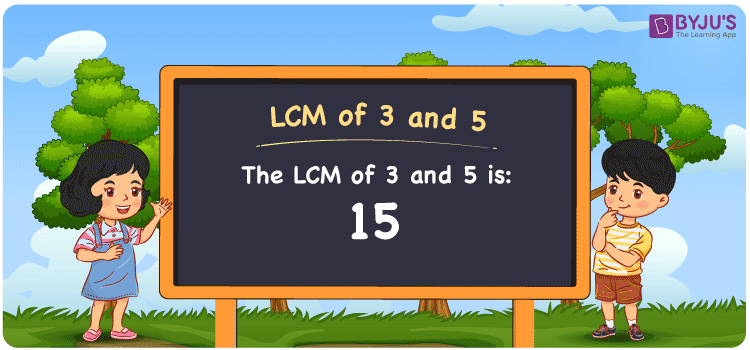Checkout JEE MAINS 2022 Question Paper Analysis : Checkout JEE MAINS 2022 Question Paper Analysis :

# LCM of 3 and 5

LCM of 3 and 5 is 15. The number which is evenly divisible by the two given numbers is the LCM. We can get the Least common multiple of 3 and 5 from the multiples which are common. The multiples of 3 are (3, 6, 9, 12, 15, 18, 21, ….) and the multiples of 5 are (5, 10, 15, 20, 25, 30, ….) respectively. The most common methods used to find the LCM are prime factorisation, division and by listing the multiples.

## What is LCM of 3 and 5?

The answer to this question is 15. The LCM of 3 and 5 using various methods is shown in this article for your reference. The LCM of two non-zero integers, 3 and 5, is the smallest positive integer 15 which is divisible by both 3 and 5 with no remainder.## How to Find LCM of 3 and 5?

LCM of 3 and 5 can be found using three methods:

• Prime Factorisation
• Division method
• Listing the multiples

### LCM of 3 and 5 Using Prime Factorisation Method

The prime factorisation of 3 and 5, respectively, is given by:

3 = 3¹

5 = 5¹

LCM (3, 5) = 15

### LCM of 3 and 5 Using Division Method

We’ll divide the numbers (3, 5) by their prime factors to get the LCM of 3 and 5 using the division method (preferably common). The LCM of 3 and 5 is calculated by multiplying these divisors.

 3 3 5 5 1 5 1 1

No further division can be done.

Hence, LCM (3, 5) = 15

### LCM of 3 and 5 Using Listing the Multiples

To calculate the LCM of 3 and 5 by listing out the common multiples, list the multiples as shown below

 Multiples of 3 Multiples of 5 3 5 6 10 9 15 12 20 15 25 18 30 21 35

LCM (3, 5) = 15

## Video Lesson on Applications of LCM## LCM of 3 and 5 Solved Examples

The LCM and GCD of two numbers are 15 and 1 respectively. If one number is 5, what is the other number?

Solution:

Consider the number as z

We know that

GCD x LCM = 5 x z

z = (GCD x LCM)/5

Substituting the values

z = (1 x 15)/5

z = 3

Hence, the other number is 3.

## Frequently Asked Questions on LCM of 3 and 5

### Find the GCF if the LCM of 3 and 5 is 15.

We know that

LCM x GCF = 3 x 5

As LCM (3, 5) is 15

15 x GCF = 15

GCF = 15/15 = 1

### Determine the LCM of 3 and 5.

The LCM of 3 and 5 is 15. To find the LCM, we first should know the multiples of 3 and 5.

Multiples of 3 = 3, 6, 9, 12, 15, 18, ……

Multiples of 5 = 5, 10, 15, 20, 25, 30, ….

The smallest multiple exactly divisible by 3 and 5 is 15.

### What is the relation between GCF and LCM of 3 and 5?

The relation between GCF and LCM of 3 and 5 is

LCM x GCF = 3 x 5

LCM x GCF = 15